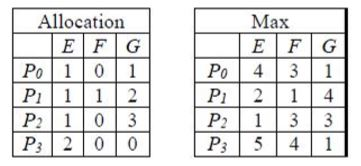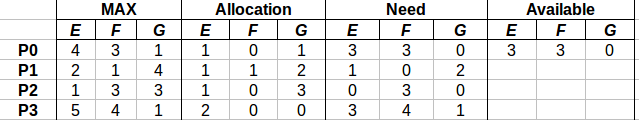GFG App
Open AppBrowser
Continue

# GATE | GATE CS 2018 | Question 55

In a system, there are three types of resources: E, F and G. Four processes P0, P1, P2 and P3 execute concurrently. At the outset, the processes have declared their maximum resource requirements using a matrix named Max as given below. For example, Max[P2, F] is the maximum number of instances of F that P2 would require. The number of instances of the resources allocated to the various processes at any given state is given by a matrix named Allocation.

Consider a state of the system with the Allocation matrix as shown below, and in which 3 instances of E and 3 instances of F are the only resources available.From the perspective of deadlock avoidance, which one of the following is true?

(A) The system is in safe state
(B) The system is not in safe state, but would be safe if one more instance of E were available
(C) The system is not in safe state, but would be safe if one more instance of F were available
(D) The system is not in safe state, but would be safe if one more instance of G were available

Explanation:Available (3, 3, 0), which can satisfy either P0 or P2.

Take P0 <3, 3, 0>. After completion we have (3, 3, 0) + (1, 0, 1) = (4, 3, 1)
Take P2 <0, 3, 0>. After completion we have (4, 3, 1) + (1, 0, 3) = (5, 3, 4)
Take P1 <1, 0, 2>. After completion we have (5, 3, 4) + (1, 1, 2) = (6, 4, 6)
Take P3 <3, 4, 1>. After completion we have (6, 4, 6) + (2, 0, 0) = (8, 4, 6)

Safe sequence : P0–>P2–>P1–>P3

Therefore, option (A) is Correct.

Quiz of this Question

My Personal Notes arrow_drop_up